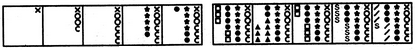# Non Verbal Reasoning - Series

Exercise : Series - Section 1
Directions to Solve

Each of the following questions consists of five figures marked A, B, C, D and E called the Problem Figures followed by five other figures marked 1, 2, 3, 4 and 5 called the Answer Figures. Select a figure from amongst the Answer Figures which will continue the same series as established by the five Problem Figures.

31.

Select a figure from amongst the Answer Figures which will continue the same series as established by the five Problem Figures.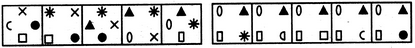(A)     (B)     (C)     (D)     (E)       (1)     (2)     (3)     (4)     (5)
1
2
3
4
5
Explanation:
All the symbols move CW half the side of the square in each step. The symbols are replaced by new ones sequentially in an ACW direction.

32.

Select a figure from amongst the Answer Figures which will continue the same series as established by the five Problem Figures.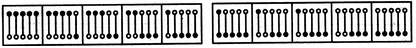(A)     (B)     (C)     (D)     (E)       (1)     (2)     (3)     (4)     (5)
1
2
3
4
5
Explanation:
One of the pins gets inverted in each step. The pins get inverted sequentially from right to left.

33.

Select a figure from amongst the Answer Figures which will continue the same series as established by the five Problem Figures.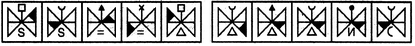(A)     (B)     (C)     (D)     (E)       (1)     (2)     (3)     (4)     (5)
1
2
3
4
5
Explanation:
The shading moves in the sequence as shown in the figure: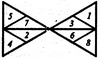The similar upper element appears in every fourth step. The lower element is replaced by a new element in every second step.

34.

Select a figure from amongst the Answer Figures which will continue the same series as established by the five Problem Figures.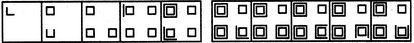(A)     (B)     (C)     (D)     (E)       (1)     (2)     (3)     (4)     (5)
1
2
3
4
5
Explanation:
Five line segments are added in each step to complete the squares in an ACW direction.

35.

Select a figure from amongst the Answer Figures which will continue the same series as established by the five Problem Figures.